Lie Algebras of Vector Fields Package - New Features in Maple 2020 - Maplesoft# Lie Algebras of Vector Fields Package

Maple 2020 includes a new package for working with Lie algebras of vector fields, the object-oriented LieAlgebrasOfVectorFields package. This leading-edge package presents a new approach to exploring properties of Lie symmetry groups.

Lie symmetry groups, when linearized about the identity symmetry, yield Lie algebras of vector fields tangent to one parameter group orbits. This linearization yields determining systems of linear homogeneous PDE (LHPDE) for the components of the Lie algebra of vector fields.

The LieAlgebrasOfVectorFields package uses differential reduction and elimination algorithms for finding both algebraic (e.g. center, lower central series, etc) and geometric (e.g. distribution, invariants, etc) properties from the determining systems of such Lie algebras of vector fields. It is based on the results in Lisle and Huang (2017) and Huang (2015).

This package is a complement to DifferentialGeometry and in particular the LieAlgebras package in DifferentialGeometry enables the flexible formulation of geometric problems arising in applications, enabling the user to formulate and solve them using exterior differential systems and moving frames while working intrinsically on the relevant manifolds.  Differential Geometry also has an extensive set of tutorials and lessons to help introduce new users to the package.

In contrast to existing approaches, the LieAlgebrasOfVectorFields package accents the early exploitation of structural, geometrical and algebraic information while delaying integration; and does utilize Maple's very strong existing pdsolve and dsolve capabilities in the integration phase when applied.  LieAlgebrasOfVectorFields gives a widened flexibility of approach, across the spectrum of integration, and structural approaches. This provides users an efficient alternative for computing symmetry structures and can be used as a preliminary analysis to determine if there exist desirable features such as large and interesting (e.g. solvable) symmetry algebras that warrants the investment of powerful more explicit solution based approaches already implemented in Maple.LAVF for Schrodinger's equation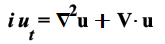with V =Here we use Schrodinger's equation of quantum mechanics in N space dimensions and one time, with a harmonic oscillator potential with time dependent coefficients to illustrate LAVF's capability with larger computations.  Such higher dimensional systems arise frequently and naturally.  It is a rich class in that it contains some simple classical PDE such as the zero potential Schrodinger equation, and also physically significant harmonic oscillator potentials, with large symmetry algebras.

The following command sequence sets the space dimension N (which the user can change if desired), and then sets up the time dependent normalized Schrodinger equation changing the form of V by changing the a(t), b(t) and c(t) (3 sets of values for a(t), b(t) and c(t) are given, and the last is the one in use by the subsequent computation).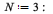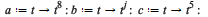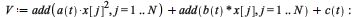Compute using PDEtools[DeterminingPDE] the determining LHPDE for the infinitesimal symmetries of the Schrodinger equation:Set up the Lie Algebra of Vector Fields for these symmetries: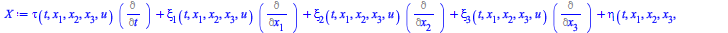We set up the lhpde object for the detEqs and its associated LAVF LX.  We then compute its dimension DimLX, Structure Constants and Lie algebra commutators: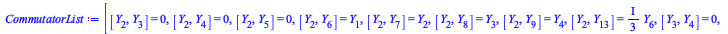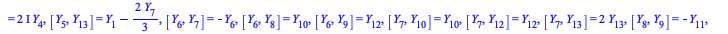"Time to compute structure=", 1.365

A laptop with I7 processor and 8 GB RAM takes about 2 secs to compute the structure for the given example with N = 3 yielding a 13 dimensional Lie algebra.  The same example takes about 100 secs for N = 10 to compute the structure for the example with N = 10 yielding a 69 dimensional Lie algebra.

Further exploratory computations are: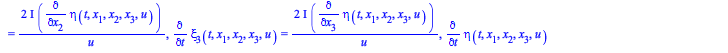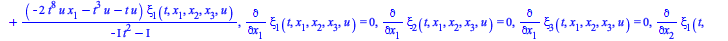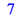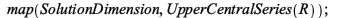IsLinearizable command

The IsLinearizable command uses LAVF to implement a remarkable algorithm introduced by Lyakhov, Gerdt and Michels (2017).

To illustrate it, consider the nonlinear ODE:We setup the vector field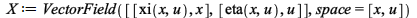and apply IsLinearizable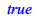Here true means that there exists an invertible change of variables that maps the nonlinear ODE to a linear ODE.  IsLinearizable is a good example of how LAVF enables the efficient computation of geometric information, in this case the existence of a linearization map, to determine if more expensive methods can be applied to a problem (in this case to determine the linearization).

Here we display the simple procedure, which uses the LAVF commands, SymmetryLAVF, SolutionDimension, DerivedAlgebra and IsAbelian.LieAlgebrasOfVectorFields:-IsLinearizable := proc(DEs::equation, X::LieAlgebrasOfVectorFields:-VectorField := NULL, \$) local n, L, m, Da, S;   1   if DEs::equation then   2       S := [DEs]       else   3       S := DEs       end if;   4   n := PDEtools[difforder](S);   5   if n < 2 then   6       return true       end if;   7   try   8       L := LieAlgebrasOfVectorFields:-SymmetryLAVF(S,X)       catch :   9       error       end try;  10   m := SolutionDimension(L);  11   if n = 2 and m = 8 then  12       return true       end if;  13   if m = n+4 then  14       return true       end if;  15   if m = n+1 or m = n+2 then  16       Da := DerivedAlgebra(L);  17       return evalb(IsAbelian(Da) and n = SolutionDimension(Da))       end if;  18   return false end proc

In the procedure above, for our example, n = 3.  SymmetryLAVF sets up the Lie algebra of vector fields, and m is the dimension of the Lie symmetry algebra m = 4 = n + 1 .  The LAVF instruction IsAbelian(Da) yields true, without determining a basis for the Derived Algebra Da.  Only algorithmic differentiations and eliminations of the determining system for Da were needed, based in an induced Lie algebra on the space of initial data for Da.

Indeed Lyakhov, Gerdt and Michels (2017) now use LAVF as an efficient precursor to their more expensive methods that use a combination of differential-elimination on the nonlinear mapping equations, combined with pdsolve to integrate their reduced form and determine the map explicitly.

Those methods yield the linearization aswhere the target linear ODE is.

References

D.A. Lyakhov, V.P. Gerdt, and D.L. Michels (2017). Algorithmic Verification of Linearizability for Ordinary Differential Equations. Proc ISSAC 2017.

I.G. Lisle and S.-L.T. Huang (2017).
Algorithmic calculus for Lie determining systems. Journal of Symbolic Computation. Vol 79 Issue P2: 482-498.

S.-L.T. Huang (2015).
Properties of Lie Algebras of Vector Fields from Lie Determining System. PhD thesis, University of Canberra.

I.G. Lisle, G.J. Reid (1998).
Geometry and Structure of Lie Pseudogroups from Infinitesimal Defining Systems. J. Symb. Comput. 26(3): 355-379.

Bereit für den nächsten Schritt?

*Die Maple-Evaluation ist für Schüler und Studenten bzw. die private Nutzung zurzeit nicht verfügbar.